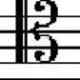#### [SOLVED] How to convert a list consisting of vector of different lengths to a usable data frame in R?

By Ico

I have a (fairly long) list of vectors. The vectors consist of Russian words that I got by using the `strsplit()` function on sentences.

The following is what `head()` returns:

``````[]
 "модно"     "создавать" "резюме"    "в"         "виде"

[]
 "ты"        "начианешь" "работать"  "с"         "этими"

[]
 "модно"            "называть"         "блогер-рилейшенз" "―"                "начинается"       "задолго"

[]
 "видел" "по"    "сыну," "что"   "он"

[]
 "четырнадцать," "я"             "поселился"     "на"            "улице"

[]
 "широко"     "продолжали" "род."
``````

Note the vectors are of different length.

What I want is to be able to read the first words from each sentence, the second word, the third, etc.

The desired result would be something like this:

``````    P1              P2           P3                 P4    P5           P6
 "модно"         "создавать"  "резюме"           "в"   "виде"       NA
 "ты"            "начианешь"  "работать"         "с"   "этими"      NA
 "модно"         "называть"   "блогер-рилейшенз" "―"   "начинается" "задолго"
 "видел"         "по"         "сыну,"            "что" "он"         NA
 "четырнадцать," "я"          "поселился"        "на"  "улице"      NA
 "широко"        "продолжали" "род."             NA    NA           NA
``````

I have tried to just use `data.frame()` but that didn't work because the rows are of different length. I also tried `rbind.fill()` from the `plyr` package, but that function can only process matrices.

I found some other questions here (that's where I got the `plyr` help from), but those were all about combining for instance two data frames of different size.#### @jgarces 2018-09-14 10:35:57

Another option could be to define a function like this (it'd mimic `rbind.fill`) or use it directly from `rowr` package:

``````cbind.fill <- function(...){
nm <- list(...)
nm <- lapply(nm, as.matrix)
n <- max(sapply(nm, nrow))
do.call(cbind, lapply(nm, function (x)
rbind(x, matrix(, n-nrow(x), ncol(x)))))
}
``````

Regards#### @andschar 2018-03-15 14:21:38

you could also use `rbindlist()` from data.table-package.

Convert vectors to data.table or data.frame and transpose it (not sure if this reduces speed a lot) with the help of `lapply()`. Then bind them with `rbindlist()` - filling missing cells with NA:

``````l = list(c("a","b","c"), c("a2","b2"), c("a3","b3","c3","d3"))
dt = rbindlist(lapply(l, function(x) data.table(t(x))),
fill = TRUE)
``````#### @akrun 2016-03-17 19:51:39

Another option is `stri_list2matrix` from `library(stringi)`

``````library(stringi)
stri_list2matrix(l, byrow=TRUE)
#    [,1] [,2] [,3] [,4]
#[1,] "a"  "b"  "c"  NA
#[2,] "a2" "b2" NA   NA
#[3,] "a3" "b3" "c3" "d3"
``````

NOTE: Data from @juba's post.

Or as @Valentin mentioned in the comments

``````sapply(l, "length<-", max(lengths(l)))
``````#### @Valentin 2018-01-26 21:47:12

I think your elegant base R solution given here is worth being mentioned as well: `sapply(l, "length<-", max(lengths(l)))`#### @Ramnath 2013-03-04 15:17:42

One liner with `plyr`

``````plyr::ldply(word.list, rbind)
``````try this:

``````word.list <- list(letters[1:4], letters[1:5], letters[1:2], letters[1:6])
n.obs <- sapply(word.list, length)
seq.max <- seq_len(max(n.obs))
mat <- t(sapply(word.list, "[", i = seq.max))
``````

the trick is, that,

``````c(1:2)[1:4]
``````

returns the vector + two NAs#### @Arun 2013-03-04 12:40:32

this could be further condensed to one line by: `sapply(word.list, '[', seq(max(sapply(word.list, length))))` (as shown here)#### @Ashe 2017-03-28 21:12:48

For those who would use @Arun's one-line solution, note that there must be a transpose `t()` to create the appropriate columns, as in the original question.#### @juba 2013-03-04 12:21:10

You can do something like this :

``````## Example data
l <- list(c("a","b","c"), c("a2","b2"), c("a3","b3","c3","d3"))
## Compute maximum length
max.length <- max(sapply(l, length))
## Add NA values to list elements
l <- lapply(l, function(v) { c(v, rep(NA, max.length-length(v)))})
## Rbind
do.call(rbind, l)
``````

Which gives :

``````     [,1] [,2] [,3] [,4]
[1,] "a"  "b"  "c"  NA
[2,] "a2" "b2" NA   NA
[3,] "a3" "b3" "c3" "d3"
``````#### @Carl Witthoft 2013-03-04 15:33:07

Aha -- what we forgot (Juba and me) is that you don't need to "fill in" the original list elements with `NA` values. The `sapply` snippet I put in a comment returns `NA` for list elements which are shorter than the requested index value. Ain't it nice of `sapply` not to crash? :-)

### [SOLVED] Convert a list to a data frame

• 2010-11-19 16:40:52
• Btibert3
• 779823 View
• 517 Score
• Tags:   r list dataframe

### [SOLVED] data.table vs dplyr: can one do something well the other can't or does poorly?

• 2014-01-29 15:21:45
• BrodieG
• 124787 View
• 765 Score
• Tags:   r data.table dplyr

### [SOLVED] Convert a list of data frames into one data frame

• 2010-05-17 17:38:24
• JD Long
• 202550 View
• 338 Score
• Tags:   list r dataframe

### how to extract data frame elements that has a specified word in R?

• 2016-03-22 18:43:23
• ashwin
• 69 View
• 0 Score